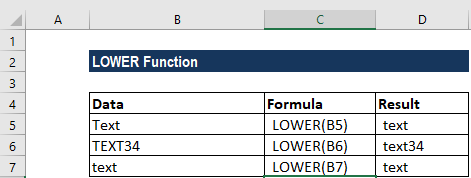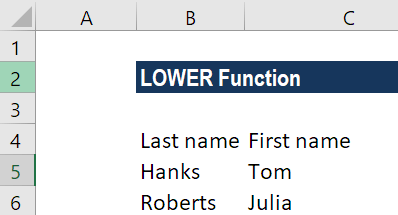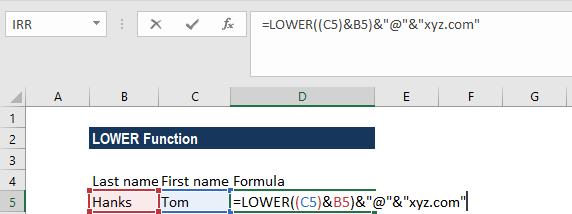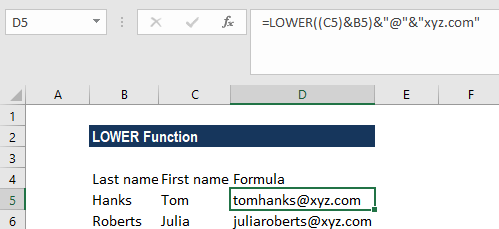# LOWER Function

Returns the lowercase version of the given text string

## What is the LOWER Function?

The LOWER function is categorized under Excel TEXT functions. The function will return the lowercase version of the text string given. It was introduced in MS Excel 2000 version and is available in all subsequent Excel versions.

In financial modeling, it can be useful in arranging data or creating data in a certain format. For example, we can create an email address from the names available in a data set.

### Formula

=LOWER(text)

This function uses one argument, that is, text. It is a required argument here. It is the text that we wish to convert to lowercase. The function will not change the characters in the text string that are not letters. So, for example, numbers and punctuations will remain unaffected when we use LOWER.

### How to use the LOWER Function in Excel?

As a worksheet function, the function can be entered as part of a formula in a cell of a worksheet. To understand the uses of the function, let us consider a few examples:

#### Example 1

Suppose we imported data from an external source and wish to convert it into lowercase.

• Text
• TEXT34
• Text

The results in Excel are given in the screenshot below:#### Example 2

Suppose we are given the first name and last name in the data set. If we wish to build email addresses using first and last names, we can use a formula that will concatenate the values. It can be done using the LOWER function. The data given are shown below:We want the email IDs to be [email protected]

The formula to use will be:We will get the results below:If we want only the first letter from the first name, we can use the LEFT function. In the above formula, the first name is joined to the last name using the concatenation operator (&), and the result is wrapped in the LOWER function, which forces all text to lower case.

Finally, the result we get is joined again to “@” and then to the domain. The domain is kept separate for convenience only.

Thanks for reading CFI’s guide to important Excel functions! By taking the time to learn and master these functions, you’ll significantly speed up your financial analysis. To learn more, check out these additional CFI resources:

• Excel Functions for Finance